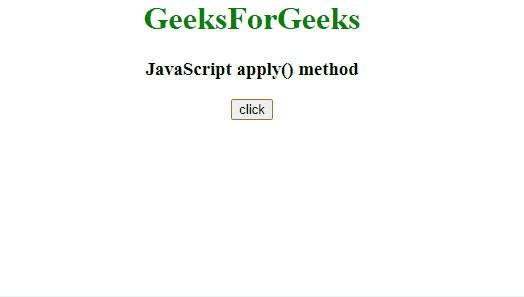GFG App
Open AppBrowser
Continue

# What is the difference between call and apply in JavaScript ?

JavaScript call() Method: It calls the method, taking the owner object as an argument. The keyword this refers to the ‘owner’ of the function or the object it belongs to. We can call a method that can be used on different objects.

Syntax:

`object.objectMethod.call( objectInstance, arguments )`

JavaScript apply() Method: The apply() method is used to write methods, which can be used on different objects. It is different from the function call() because it takes arguments as an array.

Syntax:

`object.objectMethod.apply(objectInstance, arrayOfArguments)`

Difference between the call() and apply() methods: The only difference is call() method takes the arguments separated by a comma while apply() method takes the array of arguments.

Example 1: This example uses the call() method to call a function.

## html

 `<``body` `style``=``"text-align:center;"``> ` `    ``<``h1` `style``=``"color:green;"``> ` `        ``GeeksForGeeks ` `    `` ` `    ``<``h3``>call() method ` ` `  `    ``<``button` `onClick``=``"fun()"``> ` `        ``click ` `    `` ` ` `  `    ``<``p` `id``=``"GFG"``> ` ` `  ` `  `    ``` `    ``<``script``> ` `        ``function fun() { ` `        ``let p = { ` `            ``fullName: function(addr1, addr2) { ` `            ``return this.fName + " " + this.lName ` `                    ``+ ", " + addr1 + ", " + addr2; ` `            ``} ` `        ``} ` `             `  `        ``let p1 = { ` `            ``fName:"GFGfName", ` `            ``lName: "GFGlName", ` `        ``} ` `             `  `        ``let x = p.fullName.call(p1, "India", "USA"); ` `        ``document.getElementById("GFG").innerHTML = x; ` `        ``} ` `    `` ` ``

Output:Example 2: This example does the same work by using apply() method.

## HTML

 `<``body` `style``=``"text-align:center;"``> ` ` `  `    ``<``h1` `style``=``"color:green;"``> ` `        ``GeeksForGeeks ` `    `` ` `    ``<``h3``>JavaScript apply() method ` ` `  `    ``<``button` `onClick``=``"fun()"``> ` `        ``click ` `    `` ` ` `  `    ``<``p` `id``=``"GFG"``> ` ` `  ` `  `    ``<``script``> ` `        ``function fun() { ` `            ``let p = { ` `            ``fullName: function(addr1, addr2) { ` `                ``return this.fName + " " + this.lName ` `                        ``+ ", " + addr1 + ", " + addr2; ` `            ``} ` `        ``} ` `        ``let p1 = { ` `            ``fName:"GFGfName", ` `            ``lName: "GFGlName", ` `        ``} ` `            ``let x = p.fullName.apply(p1, ["India", "USA"]); ` `            ``document.getElementById("GFG").innerHTML = x; ` `        ``} ` `    `` ` ``

Output:Let us understand differences in a tabular form -:

My Personal Notes arrow_drop_up